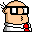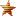Round Robin Tournament Scheduling### How many equivalence classes?

#### Richard A. DeVenezias

• Full Member
•• Posts: 45
on: September 27, 2006, 12:02:18 AM
This was posted on behalf on Maury Barbato ( sputarospo -at- alice -dot- it )

Subject: Round Robin Tournaments
Date: Wed, 20 Sep 2006 13:29:57 +0200

Hello Richard,

I'm an Italian student of Mathematics. I found on the web your very interesting pages about Round Robin Scheduling: this problem caught my attention since it seems very simple, but I soon realized that a mathematical study of this problem is quite difficult!

I posted the following problems in a sci.math thread, without receiving any answer.

Date: Sep 18, 2006 4:48 AM
Author: Maury Barbato
Subject: Round Robin Scheduling

Hello,

I'm trying to solve some problems about round robin scheduling. First of all, some definitions. In a round-robin tournament each team meets each other team exactly one time. We have 2n teams. The tournament is organized in 2n-1 rounds: in a round, each team plays exactly one match against another team. Formally, if we denote by 1,...,2n the teams, then the i-th round, 1<=i<=2n-1,is the set of all the matches R_i={{a_1,a_2},...,{a_{2n-1},a_{2n}}}, where a_i is a permutation of {1,...,2n}.

A brilliant algorithm to schedule a Round Robin tournament is given at the page http://www.nrich.maths.org/public/viewer.php?obj_id=1443&part=ind=ex&refpage=monthindex.php . Now the problems:

(I)
Given a round robin tournament R_i, i=1,...,2n-1, can you extract a match from each round, such that every team appears at the most two times in the extracted matches?
Can you find an algorithm to do it?

(II)
Consider all the possible round robin tournamentsyou can schedule with 2n teams. We say that two of these tournaments, R_i and Q_i, are equivalent if there exists a permutation s(k) of {1,...,2n} and a permutation t(k) of {1,...,2n-1} such that for every 1<=i<=2n-1, if R_t(i)={{a_1,a_2},...,{a_{2n-1},a_{2n}}}, then Q_i={{s(a_1),s(a_2)},...,{s(a_{2n-1}),s(a_{2n})}}. This is an equivalence relation. The problem is: how many equivalence classes are there?

Thank you very much for your attention. My Best Regards,
Maury

The first question can seem very strange, but it was suggested to me by a concrete problem! The second is quite difficult and I have no ideas for now. Thank you very much for your attention.